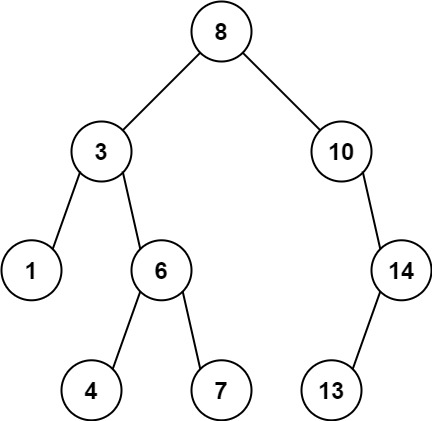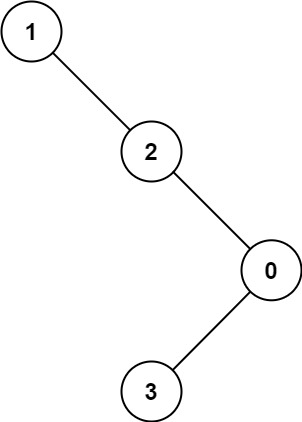1026. Maximum Difference Between Node and Ancestor

Given the `root` of a binary tree, find the maximum value `V` for which there exist different nodes `A` and `B` where `V = |A.val - B.val|` and `A` is an ancestor of `B`.

A node `A` is an ancestor of `B` if either: any child of `A` is equal to `B`, or any child of `A` is an ancestor of `B`.

Example 1:```Input: root = [8,3,10,1,6,null,14,null,null,4,7,13]
Output: 7
Explanation: We have various ancestor-node differences, some of which are given below :
|8 - 3| = 5
|3 - 7| = 4
|8 - 1| = 7
|10 - 13| = 3
Among all possible differences, the maximum value of 7 is obtained by |8 - 1| = 7.```

Example 2:```Input: root = [1,null,2,null,0,3]
Output: 3
```

Constraints:

• The number of nodes in the tree is in the range `[2, 5000]`.
• `0 <= Node.val <= 105`

1026. Maximum Difference Between Node and Ancestor
``````struct Solution;
use rustgym_util::*;

trait Preorder {
fn preorder(&self, parent: Option<(i32, i32)>, abs: &mut i32);
}

fn preorder(&self, mut parent: Option<(i32, i32)>, abs: &mut i32) {
if let Some(node) = self {
let val = node.borrow().val;
let left = &node.borrow().left;
let right = &node.borrow().right;
parent = if let Some((min, max)) = parent {
*abs = (*abs).max((min - val).abs().max((max - val).abs()));
Some((min.min(val), max.max(val)))
} else {
Some((val, val))
};
left.preorder(parent, abs);
right.preorder(parent, abs);
}
}
}

impl Solution {
fn max_ancestor_diff(root: TreeLink) -> i32 {
let mut res = 0;
root.preorder(None, &mut res);
res
}
}

#[test]
fn test() {
let root = tree!(
8,
tree!(3, tree!(1), tree!(6, tree!(4), tree!(7))),
tree!(10, None, tree!(14, tree!(13), None))
);
let res = 7;
assert_eq!(Solution::max_ancestor_diff(root), res);
}
``````